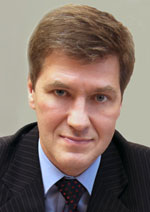Publications
Editor-in-ChiefNikiforov
D.Sc., Prof.
Partners

# TEMPERATURE DEPENDENCE CONSIDERATION ISSUE FOR COEFFICIENT OF VOLUMETRIC HEAT CAPACITY IN SIMULATION OF LASER-ARC PAD WELD PROCESS

S. Lukyanenko, V. Tretyak

Read the full article';

Abstract

The paper deals with research of steels and alloys melting and crystallization on the example of temperature field dynamics simulation for the laser-arc pad weld of composite materials. Mathematical model is one of the Stefan problems for nonlinear transient  three-dimensional Fourier equation of heat conduction with boundary conditions. The problem is solved by the finitedifference method with the pass-through calculation scheme. Nonlinearity of the problem is caused by the temperature dependency of the volumetric heat capacity coefficient that has a peak in the interval of phase transition (melting – crystallization). The linear-exponential approximation method is offered to consider this dependence. At some nodes of the discrete mesh the temperature changes from solid phase range to liquid phase at one time step on account of high heat velocity. The algorithm of temperature dependence consideration for volumetric heat capacity peak in the interval of melting – crystallization is suggested based on the nested mesh idea. Though the computational complexity is increased by suggested algorithm it provides more accurate solution and ensures account of phase transition phenomenon. Calculation results for linear and linear-exponential approximation of temperature dependence for volumetric heat capacity are compared. It is shown that in case of linear-exponential approximation the solution process on dynamic adaptive mesh is speeded up due to decreasing of time steps number.

Keywords: heat capacity equation, volumetric heat capacity coefficient, Stefan problem, pass-through calculation scheme

References
1.Лазерні технології та комп’ютерне моделювання / Під ред. Л.Ф. Головка, С.О. Лук’яненка. Київ: Вістка, 2009. 296 c.
2. LIA Handbook of Laser Materials Processing / Eds J. F. Ready, D. F. Farson. Orlando, USA: Laser Institute of America, Magnolia publishing, Inc., 2001. 715 p.
3. Князева А.Г., Крюкова О.Н., Букрина Н.В., Сорокова С.Н. Проблемы моделирования технологических процессов поверхностной обработки материалов и нанесения покрытий с использованием высокоэнергетических источников // Известия Томского политехнического университета. 2010. Т. 316. № 2. С. 93–101.
4. Третьяк В.А. Усовершенствование адаптивного метода построения сеток для задач теплопроводности с нестационарным источником энергии // Математичне та комп’ютерне моделювання. Сер. Технічні науки. 2012. В. 7. С. 197–206.
5. Picasso M., Marsden C.F., Wagniere J.D., Frenk A., Rappaz M. A simple but realistic model for laser cladding // Metallurgical and Materials Transaction B. 1994. V. 25. N 2. P. 281–291.
6. Масленников А.В., Левин Ю.Ю., Ерофеев В.А. Моделирование нестационарного формирования шва при импульсной лазерной ND:YAG-сварке // Известия Тульского государственного университета. Технические науки. 2010. В. 4. Ч. 1. С. 255–265.
7. Гончарский А.А., Туницкий В.В. Об обратной задаче синтеза оптических элементов для лазерного излучения // Вычислительные методы и программирование. М.: НИВЦ МГУ, 2006. Т. 7. С. 138–162.
8. Qi H., Mazumder J., Ki H. Numerical simulation of heat transfer and fluid flow in coaxial laser cladding process for direct metal deposition // J. Appl. Phys. 2006. V. 100. N 2. P. 024903-1–024903-11 [Электронный ресурс]. Режим доступа: http://dx.doi.org/10.1063/1.2209807, свободный. Яз. англ. (дата обращения 05.11.2013).
9. Головко Л.Ф., Лук’яненко С.О., Смаковський Д.С., Михайлова І.Ю., Агеєнко В.А. Моделювання температурного поля при зміцненні матеріалів лазерним випромінюванням // Моделювання та інформаційні технології. 2008. № 45. С. 28–35.
10. Amara E.H., Hamadi F., Achab L., Boumia O. Numerical modelling of the laser cladding process using a dynamic mesh approach // Journal of Achievements in Materials and Manufacturing Engineering. 2006. V. 15. N 1–2. P. 100–106.
11. Головко Л.Ф., Лукьяненко С.А., Смаковский Д.С., Агеенко В.А., Михайлова И.Ю. Моделирование адаптивным сеточным методом температурного поля при лазерной наплавке порошковых материалов // Электронное моделирование. 2009. Т. 31. № 1. С. 21–32.
12. Mamat M., Tofany N., Kartono A. Numerical analysis of heat conduction and phase transformation in laser transformation hardening: influences of heating duration and laser beam intensity // Applied Mathematical Sciences. 2010. V. 4. N 61–64. P. 3019–3033.
13. Мышковец В.Н., Максименко А.В., Баевич Г.А., Грищенко В.В. Термические циклы в зоне рекристаллизации при импульсной лазерной наплавке среднелегированных высокопрочных сталей